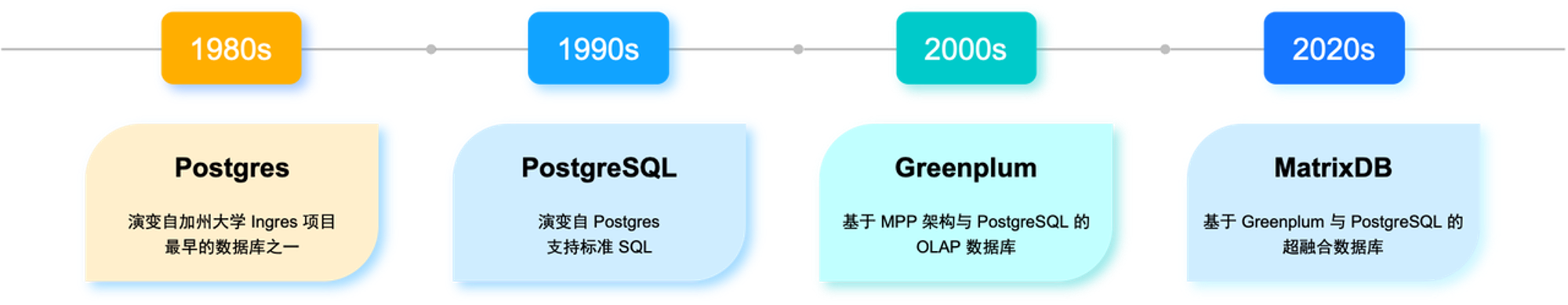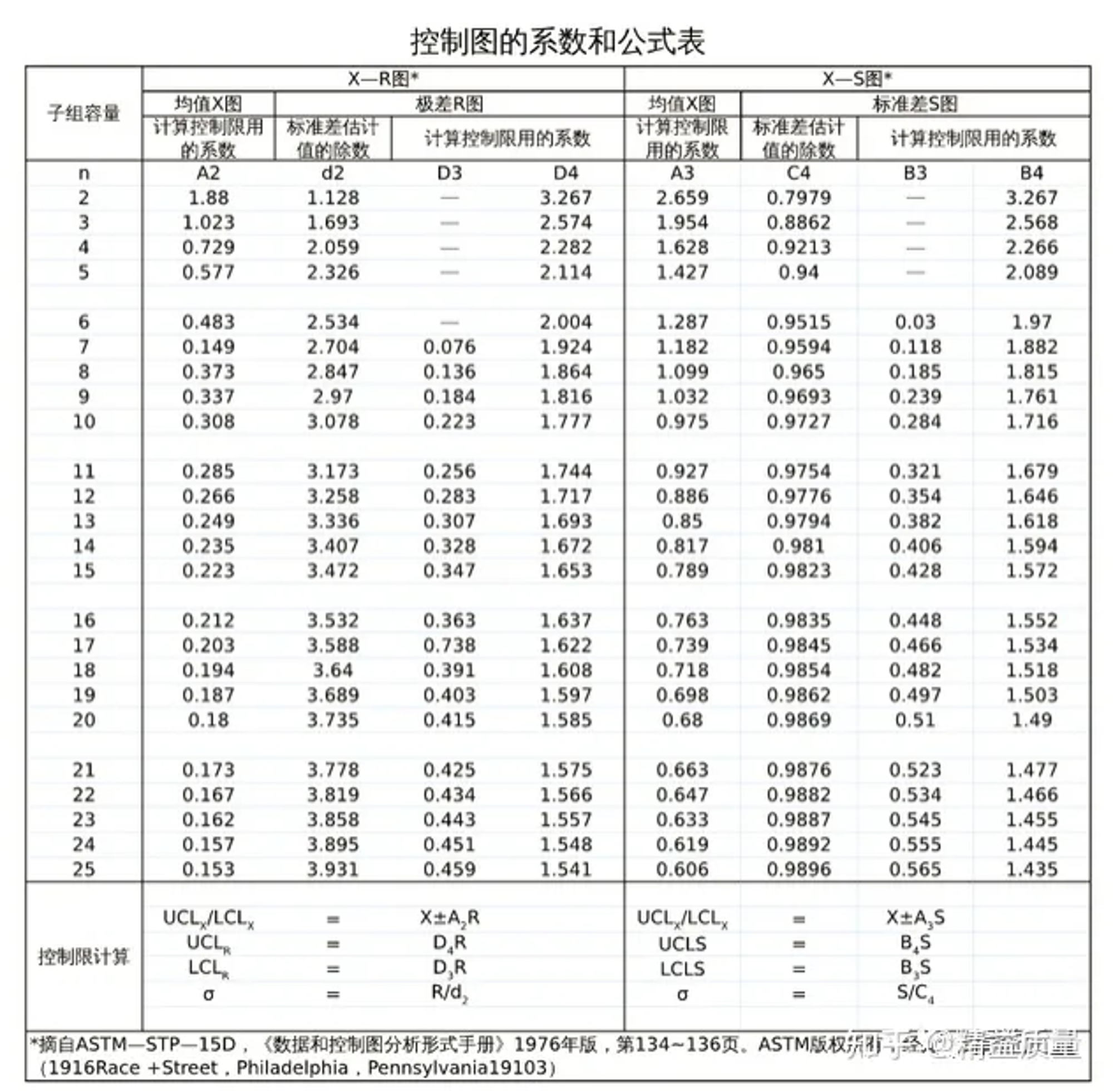f

fishgotfamous

V1

2022/12/08阅读：83主题：默认主题

# 工业过程控制

## CP、CPK与PP、PPK

CP(process capability index)PP(process performance index) 就是通过统计这个分散程度来计算出一个值，来决定和衡量生产工艺能力的。 如果过程中只包含随机因素产生的波动，这个时候使用Cp(Cpk)进行过程能力评估，Cp(Cpk)被称为短期能力指数。如果过程波动由随机因素和特殊因素共同影响而产生，那么使用Pp(Ppk)进行过程能力评估，Pp(Ppk)被称为长期能力指数。Cpk之所以被称为是短期过程能力，是因为当过程受控的情况下，在短期内，过程只会受到随机因素的影响。而Ppk之所以被称为是长期过程能力，是因为在长期情况下(比如一周，一个月等），过程会受到诸如刀具变更，设备换型，员工变更，治具调整，原材料变化等多种因素的影响，过程波动由随机因素和特殊因素共同影响而产生。CPCPKPPPPK的计算公式如下：

Cp、Cpk 中的 σ 计算公式 Pp、Ppk 中的 σ 计算公式

# 基于 YMatrix 的具体计算实现

CpCpkPpPpk 的计算方式成熟，但在大型生产企业的实际工作中，工序复杂，生产的产品众多。如何对大量工序数据进行计算，快速得到结果并实时更新成为工程师的挑战。接下来，本文将演示基于 YMatrix 超融合数据基座实现CpCpkPpPpk 的快速计算。

## 关于 YMatrix

YMatrix 传承自 PostgreSQL 与 Greenplum ，它们都是世界顶级的数据库项目。其中，PostgreSQL 在近年被Stackflow 评为最受资深程序员欢迎的数据库，没有之一，因为其强大、开放、完善的数据库功能以及良好的 SQL 标准兼容性。而 Greenplum 是基于 PostgreSQL 的 分布式数据库，在 2019 年被 Gartner 评为世界排名第三的数据仓库产品。YMatrix 基于 PostgreSQL 与 Greenplum 进行深度的内核优化，保证了生态丰富的同时，进一步提高数据库在时序、GIS 等多个领域的适应性。它内置4个高性能微内核数据引擎，完善支持标准SQL（从SQL92到SQL2016），支持多模态数据类型，包括关系数据、时序数据、GIS数据、JSON数据、文本数据、图片等；支持多模式操作，包括高并发低延迟增删改查、点查、明细查询、聚合查询、窗口查询、关联查询、多维查询、库内机器学习等。实现了一库多用，全面支持用户的数据管理需求。基于 YMatrix 的数据基座内部构造简洁、操作简便，极大的降低了客户使用数据库产品的门槛。可以让用户更关注业务的价值创造，而不是繁琐的数据性能本身。1. 示例样本 首先，我们定义样例数据为抽查精细面粉的装包重量，结果如下所示（单位：kg）：
 20.21 19.91 19.99 20.05 19.95 19.99 20.16 20.27 20.15 20.08 20.09 19.96 20.07 20.16 19.97 20.06

CREATE TABLE demo_normal(value float,group int);INSERT INTO demo_normal VALUES                            (20.21,1),(19.91,1),(19.99,1),(20.05,1),                            (19.95,1),(19.99,1),(20.16,1),(20.27,1),                            (20.15,1),(20.08,1),(20.09,1),(19.96,1),                            (20.07,1),(20.16,1),(19.97,1),(20.06,1);
1. 计算累计分布 计算公式中的概率分布函数，由于 Python 比较方便做概率密度的计算，此处引入 plpython 的自定义函数实现，具体如下：
-- 函数注册DROP FUNCTION if exists normcdf(x double precision,loc double precision,scale double precision);create or replace function normcdf(x double precision,loc double precision,scale double precision)returns double precision as$$from scipy.stats import normreturn norm.cdf(x,loc=loc,scale=scale)$$language plpython3u immutable strict;-- 查询使用with mean_and_std as (select avg(value) as mean,stddev(value) as std from demo_normal)select    value,    normcdf(value,mean,std) as fxifrom demo_normal CROSS JOIN mean_and_std order by value;

with    mean_and_std as     (select avg(value) as mean,stddev(value) as std from demo_normal),    norm_cdf as     (select value,            normcdf(value,mean,std) as fxi,            row_number() over (order by value) as rn,            row_number() over (order by value desc ) as rnd     from demo_normal CROSS JOIN mean_and_std order by value)select    nc1.value,    nc1.fxi,    nc2.mfxi as mfxidfrom norm_cdf nc1 left join (select rnd,1-fxi as mfxi from norm_cdf) nc2 on nc1.rn = nc2.rnd;

with    mean_and_std as     (select avg(value) as mean,            stddev(value) as std,            count(value) as number_value      from demo_normal),    norm_cdf as     (select value,            number_value,            normcdf(value,mean,std) as fxi,            row_number() over (order by value) as rn,            row_number() over (order by value desc ) as rnd     from demo_normal CROSS JOIN mean_and_std order by value),    fxi_mfxid as     (select nc1.value,            nc1.number_value,            nc1.rn,            nc1.fxi,            nc2.mfxi as mfxid     from norm_cdf nc1 left join      (select rnd,1-fxi as mfxi from norm_cdf) nc2      on nc1.rn = nc2.rnd)select value ,(2*rn-1)*(ln(fxi)+ln(mfxid)) as s_for_sum from fxi_mfxid;

with    mean_and_std as     (select avg(value) as mean,stddev(value) as std,count(value) as number_value from demo_normal),    norm_cdf as     (select value,            number_value,            normcdf(value,mean,std) as fxi,            row_number() over (order by value) as rn,            row_number() over (order by value desc ) as rnd     from demo_normal CROSS JOIN mean_and_std order by value),    fxi_mfxid as     (select nc1.value,            nc1.number_value,            nc1.rn,            nc1.fxi,            nc2.mfxi as mfxid     from norm_cdf nc1 left join (select rnd,1-fxi as mfxi from norm_cdf) nc2 on nc1.rn = nc2.rnd),    for_sum as     (select (2*rn-1)*(ln(fxi)+ln(mfxid)) as s_for_sum from fxi_mfxid)select -1*count(s_for_sum) - sum(s_for_sum)/count(s_for_sum) as AD_value  from for_sum;

with    mean_and_std as     (select avg(value) as mean,stddev(value) as std,count(value) as number_value from demo_normal),    norm_cdf as     (select value,            number_value,            normcdf(value,mean,std) as fxi,            row_number() over (order by value) as rn,            row_number() over (order by value desc ) as rnd     from demo_normal CROSS JOIN mean_and_std order by value),    fxi_mfxid as     (select nc1.value,            nc1.number_value,            nc1.rn,            nc1.fxi,            nc2.mfxi as mfxid     from norm_cdf nc1 left join (select rnd,1-fxi as mfxi from norm_cdf) nc2 on nc1.rn = nc2.rnd),    for_sum as     (select number_value ,(2*rn-1)*(ln(fxi)+ln(mfxid)) as s_for_sum      from fxi_mfxid),    ad as     (select -1*count(s_for_sum) - sum(s_for_sum)/count(s_for_sum) as AD_value      from for_sum)select case    when AD_value >= 0.6 then exp(1.2937-5.709*AD_value+0.0186*AD_value*AD_value)    when 0.34 < AD_value and AD_value < 0.6 then exp(0.9177-4.279*AD_value-1.38*AD_value*AD_value)    when 0.2 < AD_value and AD_value <= 0.34 then 1-exp(-8.318+42.796*AD_value-59.938*AD_value*AD_value)    when AD_value <= 0.2 then 1-exp(-13.436+101.14*AD_value-223.73*AD_value*AD_value)    end as P_valufrom ad;

## CP、CPK、Pp、PpK 计算实现

1. 样本导入 有一个产品的某个尺寸的公差为5.33±0.05，每20分钟从产线上拿取一个产品进行测量，收集了30个数据，求Cp和Pp。（每次拿取一个产品可视为子组数为1）

No. Data No. Data No. Data No. Data No. Data
1 5.343 7 5.304 13 5.314 19 5.308 25 5.280
2 5.326 8 5.322 14 5.287 20 5.279 26 5.347
3 5.307 9 5.297 15 5.321 21 5.316 27 5.329
4 5.325 10 5.326 16 5.289 22 5.361 28 5.301
5 5.302 11 5.316 17 5.299 23 5.319 29 5.313
6 5.313 12 5.311 18 5.314 24 5.283 30 5.366
2. 示例数据入库

DROP TABLE IF EXISTS demo_cp_pp;CREATE TABLE demo_cp_pp(value float,order_id int);INSERT INTO demo_cp_pp VALUES    (5.343,1),(5.326,2),(5.307,3),(5.325,4),(5.302,5),    (5.313,6),(5.304,7),(5.322,8),(5.297,9),(5.326,10),    (5.316,11),(5.311,12),(5.314,13),(5.287,14),(5.321,15),    (5.289,16),(5.299,17),(5.314,18),(5.308,19),(5.279,20),    (5.316,21),(5.361,22),(5.319,23),(5.283,24),(5.280,25),    (5.347,26),(5.329,27),(5.301,28),(5.313,29),(5.366,30);
1. AD验证 通过前文方法，对目标表进行AD验证，注意将SQL语句中目标表改成demo_cp_pp，得到P值为0.3268451871113953，大于0.05，目标样本符合正态分布。
2. Cpσ 计算 让我们回顾Cpσ 的计算公式：

子组数量大于1

子组数量为1

其中的d2来自于控制图系数表，d2=1.128本示例子组数量为1，取第二个公式，其中 MR 值为移动极差均值，即对于每一列后一项减去前一项的绝对值再去平均：

select avg(mr_value)/1.128 as sigma from (select value,       abs(lag(value) over (order by order_id) - demo_cp_pp.value) as mr_valuefrom demo_cp_pp)mr_cal;

select std_dev(value) from demo_cp_pp;

select (5.38-5.28)/(6*cp_sigma) as cp,       (5.38-5.28)/(6*pp_sigma) as pp,       LEAST((5.38-miu)/(3*cp_sigma),(miu-5.28)/(3*cp_sigma)) as cpk,       LEAST((5.38-miu)/(3*pp_sigma),(miu-5.28)/(3*pp_sigma)) as ppkfrom (select avg(mr_value)/1.128 as cp_sigma,       stddev(value) as pp_sigma,       avg(value) as miu      from (select value,                   abs(lag(value) over (order by order_id) -                        demo_cp_pp.value) as mr_value            from demo_cp_pp)mr_cal)sigma_cal;

## 持续监测

1. 目标工序事实表：
CREATE table public.ppk_demo_target(    station character varying(128),    slot character varying(128),    low_limit double precision,    hi_limit double precision,    result_val double precision);ALTER table public.ppk_demo_target    OWNER TO mxadmin;

drop VIEW if exists ppk_demo_view;CREATE VIEW ppk_demo_view WITH (CONTINUOUS) ASselect station,       slot,       stddev_samp(result_val) as sigma,       avg(result_val) as miu,       min(low_limit) as LSL,       max(hi_limit) as USLfrom ppk_demo_target GROUP BY station,slot order by station,slot;
1. 查询时通过视图中的μσ来完成PpPpk的计算。
select (USL-LSL)/(6*sigma) as pp,       LEAST((USL-miu)/(3*sigma),(miu-LSL)/(3*sigma)) as ppkselect * from ppk_demo_view order by station,slot;

f
V1